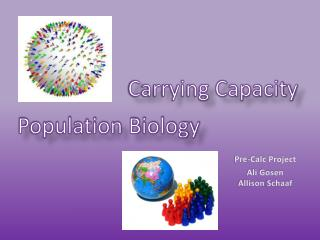DownloadDownload PresentationCarrying Capacity

# Carrying Capacity

Download Presentation## Carrying Capacity

- - - - - - - - - - - - - - - - - - - - - - - - - - - E N D - - - - - - - - - - - - - - - - - - - - - - - - - - -
##### Presentation Transcript

1. Carrying Capacity Population Biology Pre-Calc Project Ali Gosen Allison Schaaf

2. Our Table of Populations The Source:

3. Linear Equation Equation: Y=2.5682x-1220 Prediction for 2030 based off of Equation: 3,993,446,000 people R for this equation: 0.586003

4. Exponential Equation (our pick) Equation: Y=105.22e0.0017x Prediction for 2030 from this equation: 3,317,787,408 R for this equation: 0.837854

5. Logarithmic Equation Equation: Y=7276.5ln(x)-51268 Prediction for 2030 based on equation: 4,148,303,736 R for this equation: 0.568770604

6. Carrying Capacity and Population Biology Carrying Capacity: the Earth’s “maximum load”, can not be determined as one fixed number. The determination factor of capacity is the environment. The carrying capacity depends on food, habitat, water, and other necessities available in the environment. Population Biology: the study of populations of organisms and the regulation of population size In the field of population biology, scientists use evolution, ecology, systematics, genetics, mathematics, and statistics to figure out the interactions of humans and the environment.